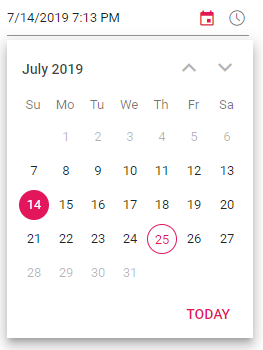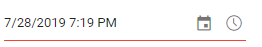# DateTime Range in Blazor Datetime Picker Component

15 Dec 20221 minute to read

DateTimePicker provides an option to select a date and time value within a specified range by using the Min and Max properties. The Min value must always be less than the Max value.

The `Value` property depends on the Min/Max with respect to StrictMode property. For more information about StrictMode, refer to the Strict Mode section from the documentation.

The following code allows selecting a date within the range from 7th to 27th day in a month.

``````@using Syncfusion.Blazor.Calendars

<SfDateTimePicker TValue="DateTime?" Min='@MinDateTime' Max='@MaxDateTime' Value='@DateTimeValue'></SfDateTimePicker>

@code {
public DateTime MinDateTime {get;set;} = new DateTime(DateTime.Now.Year,DateTime.Now.Month, 7, 0, 0, 0);
public DateTime MaxDateTime {get;set;} = new DateTime(DateTime.Now.Year, DateTime.Now.Month, 27, DateTime.Now.Hour, DateTime.Now.Minute, DateTime.Now.Second);
public DateTime? DateTimeValue {get;set;} = new DateTime(DateTime.Now.Year, DateTime.Now.Month, 14, DateTime.Now.Hour, DateTime.Now.Minute, DateTime.Now.Second);
}``````When the Min and Max properties are configured and the selected datetime value is out-of-range or invalid, then the model value will be set to `out of range` datetime value or `null` respectively with highlighted `error` class to indicate the datetime is out of range or invalid.

``````@using Syncfusion.Blazor.Calendars

<SfDateTimePicker TValue="DateTime?" Min='@MinDateTime' Max='@MaxDateTime' Value='@DateTimeValue'></SfDateTimePicker>

@code {
public DateTime MinDateTime {get;set;} = new DateTime(DateTime.Now.Year,DateTime.Now.Month, 7, 0, 0, 0);
public DateTime MaxDateTime {get;set;} = new DateTime(DateTime.Now.Year, DateTime.Now.Month, 27, DateTime.Now.Hour, DateTime.Now.Minute, DateTime.Now.Second);
public DateTime? DateTimeValue {get;set;} = new DateTime(DateTime.Now.Year, DateTime.Now.Month, 28, DateTime.Now.Hour, DateTime.Now.Minute, DateTime.Now.Second);
}``````NOTE

If the value of `Min` or `Max` properties changed through code behind, you have to update the `Value` property to set within the range.